#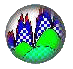Interactive Real Analysis - part of MathCS.org

Next | Previous | Glossary | Map | Discussion

## 6.1. Limits

We now want to combine some of the concepts that we have introduced before: functions, sequences, and topology. In particular, if we have some function f(x) and a given sequence { an }, then we can apply the function to each element of the sequence, resulting in a new sequence. What we would want is that if the original sequence converges to some number L, then the new sequence { f( an )} should converge to f(L), and if the original sequence diverges, the new one should diverge also. This seems not too much to ask for, but is quite simple minded.
 Example 6.1.1: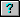Consider the function f, where f(x) = 1 if x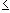0 and f(x) = 2 if x > 0. The sequence { 1/n } converges to 0. What happens to the sequence { f( 1/n ) } ? The sequence { 3 + (-1)n } is divergent. What happens to the sequence { f ( 3 + (-1)n ) } ? The sequence { (-1)n / n } converges to zero. What happens to the sequence { f ( (-1)n / n ) } ?
As the above easy example shows, things can be more complicated than anticipated. Therefore, we have to attack the problem more systematically. First, we need to define what we mean by 'limit of a function'.
 Definition 6.1.2: Limit of a Function (sequences version) A function f with domain D in R converges to a limit L as x approaches a number c if D - {c} is not empty and for any sequence { xn in D - {c} that converges to c the sequence { f ( xn ) } converges to L. We writef(x) = L
 Examples 6.1.3:Apply this definition in these cases: Let f(x) = m x + b. Then does the limit of that function exist at an arbitrary point x ? Let g(x) = [x], where [x] denotes the greatest integer less than or equal to x. Then does the limit of g exist at an integer ? How about at numbers that are not integers ? In the above definition, does c have to be in the domain D of the function ? Is c in the closure(D) ? Do you know a name for c in terms of topology ?
The above definition works quite well to show that a function is not continuous, because you only have to find one particular sequence whose images do not converge as a sequence. It is not a good definition, in general, to prove convergence of a function, because you will have to check every possible convergent sequence, and that is hard to do. We would therefore like another definition of convergence or limit of a function.
 Definition 6.1.4: Limit of a function (epsilon-delta Version) A function f with domain D in R converges to a limit L as x approaches a number cclosure (D) if: given any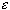> 0 there exists a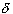> 0 such that: if xD and | x - c | <then | f(x) - L | <Example 6.1.5:Consider the function f with f(x) = 1 if x is rational and f(x) = 0 if x is irrational. Does the limit of f(x) exist at an arbitrary number x ?
Regardless of which of the two definitions might be considered easier to use in a particular situation, the basic problem right now is that we have two different definitions for the same concept. We therefore have to show that both definitions are actually equivalent to each other.
 Proposition 6.1.6: Equivalence of Definitions of Limits If f is any function with domain Din R, and cclosure(D) then the following are equivalent: For any sequence { xn } in D that converges to c the sequence { f ( xn ) } converges to L given any> 0 there exists a> 0 such if xclosure(D) and | x - c | <then | f(x) - L | <In other words, both definitions of continuity are equivalent, and we can use which ever seems the easiest. Here are some basic properties of limits of functions.
 Proposition 6.1.7: Properties for limits of Functions Iff(x) exists, the limit is unique.[ f(x) + g(x) ] =f(x) +g(x), provided thatf(x) andg(x) exist.[ f(x) g(x) ] =f(x)g(x), provided thatf(x) andg(x) exist.[ f(x) / g(x) ] =f(x) /g(x), provided thatf(x) andg(x) exist andg(x) # 0.
Sometimes a function may not have a limit using the above definitions, but when the domain of the function is restricted, then a limit exists. This leads to the concepts of one-sided limits.
 Definition 6.1.8: One-Sided Limits of a Function If f is a function with domain D and cclosure(D). Then: f has a left-hand limit L at c if for every> 0 there exists> 0 such that if xD and c -< x < c then | f(x) - L | <. We write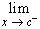f(x) = L. f has a right-hand limit L at c if for every> 0 there exists> 0 such that if xD and c < x < c +then | f(x) - L | <. We write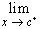f(x) = L.
This is the formal definition of x approaching c either only from the right side, or only from the left side. These one-sided limits are related to regular limits in a straight forward manner:
 Proposition 6.1.9: Limits and One-Sided Limitsf(x) = L if and only iff(x) = L andf(x) = L
Now that we have some idea about limits of functions, we will move to the next question: if some sequence converges to c, and the function converges to L as x approaches c, then when is it true that f(c) = L ? This will be the contents of the next section, continuity.
Next | Previous | Glossary | Map | Discussion